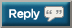# Thread: Let's OHP 2,020,000 lbs in 2020

1. + 1,320 = 1,651,8952. 3,212 + 1,651,895 = 1,655,1073. + 2060 = 1,657,1674. + 900 = 1,658,0675.6. 7 x 42.5kg
7 x 42.5kg
7 x 42.5kg
8 x 42.5kg (AMRAP)

29 x 42.5kg = 1232.5kg (2717lb)

2717 + 1,658,067 = 1,660,7847. 7 x 42.5kg
7 x 42.5kg
7 x 42.5kg
8 x 42.5kg (AMRAP)

29 x 42.5kg = 1232.5kg (2717lb)

2717 + 1,658,067 = 1,660,784
2,200 + 1,660,784 = 1,662,9848. 1,662,984 + 3,278 = 1,666,2629. + 1,470 = 1,667,732 That's all she wrote until next week.10. + 1,470 = 1,667,732 That's all she wrote until next week.3,487 + 1,667,732 = 1,671,21911. + 1980 = 1,673,19912. + 1865 = 1,675,06413.14. + 1,100 = 1,678,864
That's it for the week, methinks.15. + 1,100 = 1,678,864
That's it for the week, methinks.
+ 2060 = 1,680,92416. I've switched to doing a strict/military press, hence my weights are reduced. Last set is an AMRAP, followed by push press until I'm at RPE10.

7 x 35kg
7 x 35kg
7 x 35kg
7 x 35kg + 5 x 35kg

33 x 35kg = 1155kg (2546lb)

2546 + 1,680,924 = 1,683,47017. I've switched to doing a strict/military press, hence my weights are reduced. Last set is an AMRAP, followed by push press until I'm at RPE10.

7 x 35kg
7 x 35kg
7 x 35kg
7 x 35kg + 5 x 35kg

33 x 25kg = 1155kg (2546lb)

2546 + 1,680,924 = 1,683,470
+3,418 = 1,686,88818. 70kg/155lbs x5 = 775
70kg/155lbs x5 = 775
70kg/155lbs x5 = 775
60kg/130lbs x10 = 1300

775x3 = 2325

2325 +1300 = 3325

3325+1 686 888 = 1 690 21319. 70kg/155lbs x5 = 775
70kg/155lbs x5 = 775
70kg/155lbs x5 = 775
60kg/130lbs x10 = 1300

775x3 = 2325

2325 +1300 = 3325

3325+1 686 888 = 1 690 213
+ 2060 = 1,692,27320. +225=1,692,498
Not much but I started my 5x5 program today. Started with the bar since I have not lifted in years. Really had to swallow my pride doing everything with the empty bar.21. +225=1,692,498
Not much but I started my 5x5 program today. Started with the bar since I have not lifted in years. Really had to swallow my pride doing everything with the empty bar.
Hey man just checking you're calculating right. If you're doing 5x5 it should be the bar weight x 25. This would mean the bar you are using weighs 9lb, is that correct?

+2,221 = 1,694,71922. 2,240 + 1,694,719 = 1,694,71923. Correction - New total after your 2,240 should be 1,696,959

+2,970 = 1,699,92924. Hey man just checking you're calculating right. If you're doing 5x5 it should be the bar weight x 25. This would mean the bar you are using weighs 9lb, is that correct?

+2,221 = 1,694,719
Lol yeah man I calculated 1 set not 5. Will do better next go around. Good eye.25. Lol yeah man I calculated 1 set not 5. Will do better next go around. Good eye.
Thought that might be the case, just add on 900lb to the current total and you're set26. Correction - New total after your 2,240 should be 1,696,959

+2,970 = 1,699,929
Took a de-training week and forgot to add my total when I re-started on Monday so 2 days worth here...

1,699,929 + 6,248 = 1,706,17727. Took a de-training week and forgot to add my total when I re-started on Monday so 2 days worth here...

1,699,929 + 6,248 = 1,706,177
+2900 = 1,708,07728.29. 3212 + 1,714,377 = 1,717,58930. + 2750 = 1,720,339

Hallelujah the gyms are now open in the England!!#### Posting Permissions

• You may not post new threads
• You may not post replies
• You may not post attachments
• You may not edit your posts